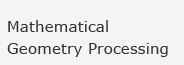Home » Integral Curves

## Type and Study Integral Curves

Here JavaView is used as an interface for Mathematica`s NDSolve.

Pick and drag the initial point of the differential equation in the viewer window. This modifies the initial `x` and `y` values.

Type a new differential equation in the syntax of Mathematica.

Press Evaluate to solve the equation with the current parameter settings.

Please be patient, the server needs some time to send the data.

Controls in ODE project panel:

 ODE text field Type a new ordinary differential equation in Mathematica syntax. Text field "Order" Defines the order (highest derivative of `y`) of the differential equation Slider "Plot Points" Number of Points for the Plot. Slider "Length" Length of the `x`-interval to evaluate the differential equation. Slider "Initial x" Minimum value for `x`-interval Text fields "Initial y" The first text field shows the initial `y`-value, the second text field shows the initial value of the first derivative of `y`, if "Order" is greater than 2, further text fields for the higher derivatives are displayed.
© 2006 Freie Universität Berlin | Feedback |
Stand: 21.04.2008